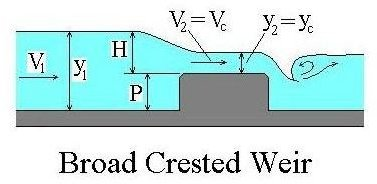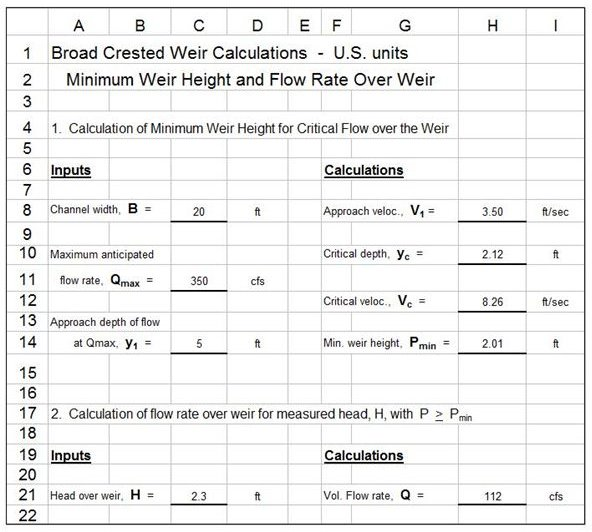# Broad Crested Weir Flow Rate Calculations for Open Channel Flow Measurement with Excel Spreadsheets

Page content

Image Credit: Middle Rio Grande Conservancy District

## Equations for Broad Crested Weir Flow Rate Calculations

The equation for calculating broad crested weir flow rate is quite straight forward: Q = 1.6 L H3/2,where Q is the flow rate in cfs, L is the length of the weir in ft, and H is the head over the weir (as shown in the diagram at the right) in ft. This simple equation comes with a caveat however. There must be critical flow over the weir crest in order for this equation to be used.

The four following equations, based on fundamental open channel flow principles, can be used to calculate the minimum weir height, P (see the diagram), needed to ensure critical flow over the weir crest. The four equations are:

1. y1 + V12/2g = y2 + P + V22/2g, an expression of the energy equation, using variables shown in the diagram. P, y1, and y2 are in ft, V1 and V2 are in ft/s, g = 32.17 ft/sec2.
2. V1 = Q/y1B, from the definition of average velocity in an open channel, assuming that the channel is approximately rectangular in cross-section. B is the width of the channel, typically equal to the weir length, L.
3. Vc = Q/ycB - This is the same as the previous equation, but for the flow over the weir crest.
4. yc = [Q2/gB2]1/3, based on the fact that the specific energy is a minimum for critical flow conditions.

The Broad Crested Weir Article mentioned above gives more detail on these equations and an example calculation of P for specified values of Q, y1, and B.The image at the left shows an Excel spreadsheet that can be used to calculate the minimum broad crested weir height needed for critical flow, with specified channel width, B, maximum anticipated flow rate, Qmax, and approach depth of flow, y1. The Excel formulas will also calculate the open channel flow rate for a specified head over the weir, H, assuming that critical flow takes place over the weir.

The Excel spreadsheet shown and the equation given are for U.S. units. The four equations given for calculating P remain the same for S.I. units, with flow rate in m3/s, velocities in m/s, depths and widths in m, and g = 9.81 m/s2. The equation for flow rate becomes: Q = 0.886 L H3/2, with Q in m3/s, and L and H in m. The spreadsheet shown is available in either U.S. or S.I. units through the following links.